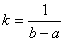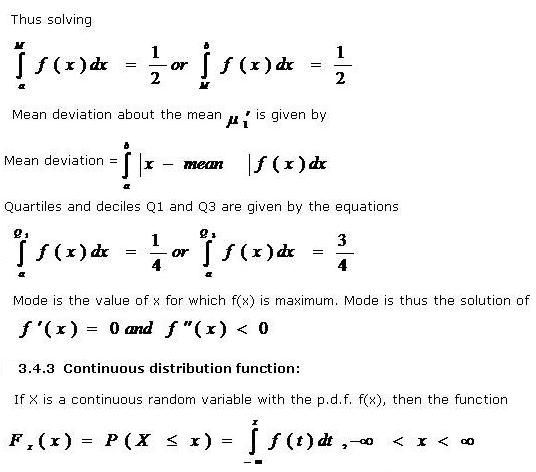# Statistics Assignment Help With Continuous Random Variable

A random variable X is said to be continuous if it can take all possible alues between certain limits. In other words, a random variable is said to be continuous when its different values cannot be put in one to one correspondence with a set of positive integers.

A continuous random variable is a random variable that can be measured to any desired degree of accuracy. Examples of continuous random variables are age, height etc.

## 3.4.1 Probability density function:

Consider the small interval (x,x+dx) of length dx round the point x. let f(x) be any continuous function of x so that f(x) dx represents the probability that x falls in the infinitesimal interval (x, x+dx).Symbolically

P(x≤X≤x+dx) =fx(x)dx

The function fx(x) so defined is known as probability density function or simply density function of random variables X and is usually abbreviated as p.d.f. the expression, f(x) dx, usually written as dF(x), is known as the probability differential and the curve y = f(x) is known as the probability density curve or simply probability curve.

The properties of the probability density function (p.d.f) of a random variable X usually denoted by fx(x) or simply by f(x) is

• (i) f(x) ≥ 0, -∞ < x < ∞
• (ii)• (iii) The probability P(E) given byis well defined for any event E.

### 3.4.2 Various measures of central tendency, dispersion, skewness and kurtosis for continuous probability distribution:

The formula for these measures in case of discrete frequency distribution can be easily extended to the case of continuous probability distribution by simply replacing pi = fi/N by f(x) dx, xi by x and the summation over ‘i’ by integration over the specified range of the variable X.

Let fx(x) or f (x) be the p.d.f of a random variable X where X is defined from a to b. then

###is called the distribution function (d.f) or sometimes, the cumulative distribution function(c.d.f.) of the random variable X.